# Class 12 Maths NCERT Solutions for Chapter 8 Application of Integrals Exercise 8.2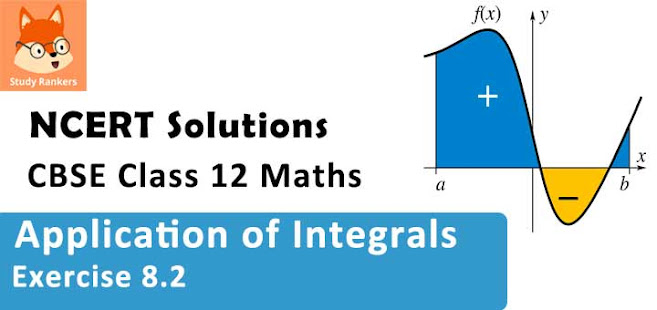### Integrals Exercise 8.2 Solutions

1. Find the area of the circle 4x2 + 4y2 = 9 which is interior to the parabola x2 = 4y

Solution

The required area is represented by the shaded area OBCDO.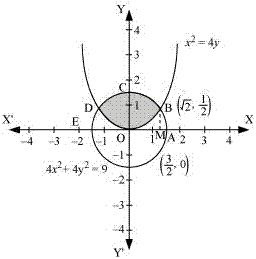Solving the given equation of circle, 4x2 + 4y2 = 9, and parabola, x2 = 4y , we obtain the point of intersection as B(√2, 1/2) and D(-√2, 1/2).
It can be observed that the required area is symmetrical about y - axis.
Area OBCDO = 2 × Area OBCO
We draw BM perpendicular to OA.
Therefore, the coordinates of M are (√2, 0).
Therefore, Area OBCO = Area OMBCO - Area OMBO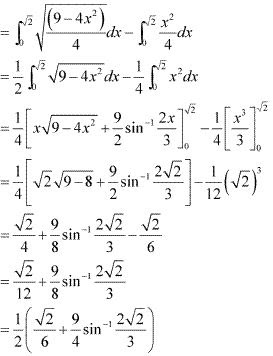Therefore, the required area OBCDO is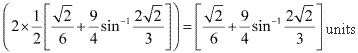2. Find the area bounded by curves (x – 1)2 + y2 = 1 and x2 + y 2 = 1

Solution

The area bounded by the curves, (x - 1)2 + y2 = 1 and x2 + y2 = 1, is represented by the shaded area as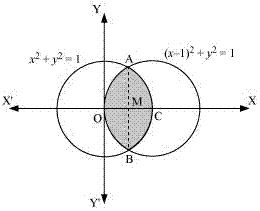On solving the equations, (x - 1)2 + y2 = 1 and x2 + y2 = 1, we obtain the point of intersections as A (1/2, √3/2) and B(1/2, √3/2)
It can be observed that the required area is symmetrical about x - axis.
∴ Area OBCAO = 2 × Area OCAO
We join AB, which intersects OC at M, such that AM is perpendicular to OC.
The coordinates of M are (1/2, 0)
⇒ Area OCAO = Area OMAO + Area MCAM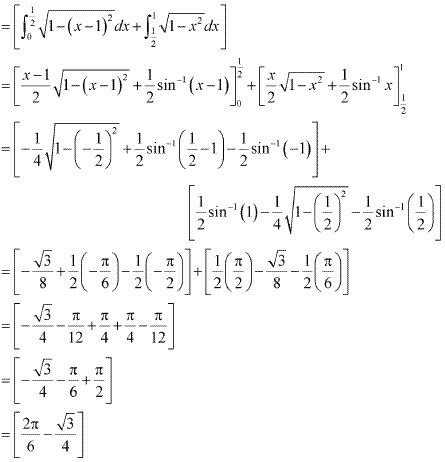Therefore, required area OBCAO = 2 × [2π/6 - √3/4) = (2π/3 - √3/2) units

3. Find the area of the region bounded by the curves y = x+ 2, xx = 0 and x = 3

Solution

The area bounded by the curves, y = x+ 2, xx = 0 and x = 3, is represented b the shaded area OCBAO as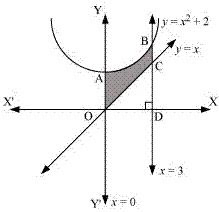Then, Area OCBAO = Area ODBAO - Area ODCO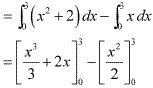= (9 + 6) - (9/2)
= 15 - (9/2)
= 21/2 units

4. Using integration finds the area of the region bounded by the triangle whose vertices are (–1, 0), (1, 3) and (3, 2).

Solution

BL and CM are drawn perpendicular to x - axis.
It can be observed in the following figure that,
Area (Δ ACB) = Area (ALBA) + Area (BLMCB) - Area (AMCA) ...(1)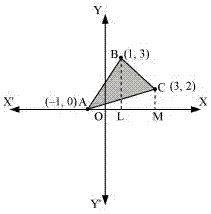Equation of line segment AB is
y - 0 = [(3 - 0)/(1 + 1)] (x + 1)
y = 3/2 (x + 1)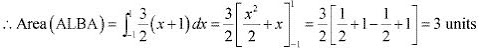Equation of line segment BC is
y - 3 = [(2 - 3)/(3 - 1)](x - 1)
y = 1/2 (-x + 7)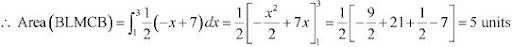Equation of line segment AC is
y - 0 = [(2 -0)/(3 + 1)] (x + 1)
y = 1/2 (x + 1)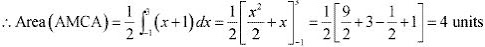Therefore, from equation (1), we obtain
Area (ΔABC) = (3 + 5 - 4) = 4 units

5. Using integration find the area of the triangular region whose sides have the equations y = 2x +1, y = 3x + 1 and = 4.

Solution

The equations of sides of the triangle are y = 2x + 1, y = 3x + 1, and x = 4
On solving these equations, we obtain the vertices of triangle as A(0, 1), B(4, 13), and C(4, 9)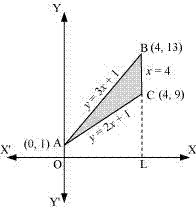It can be observed that,
Area (∆ACB) = Area (OLBAO) - Area (OLCAO)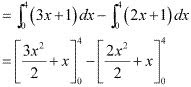= (24 + 4) - (16 + 4)
= 28 - 20
= 8 units

6. Smaller area enclosed by the circle x2 + y2 = 4 and the line x + y = 2 is
A. 2 (π – 2)
B. π – 2
C. 2π – 1
D. 2 (π + 2)

Solution

The smaller area enclosed by the circle, x2 + y2 = 4 and the line x + y = 2 , is represented by the shaded area ACBA as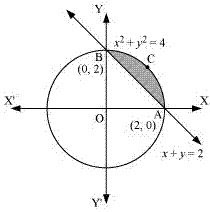It can be observed that,
Area ACBA = Area OACBO - Area (Δ OAB)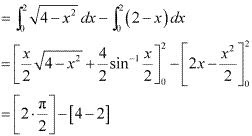= (π - 2) units
Thus, the correct answer is B.

7. Area lying between the curve y2 = 4x and y = 2x is
A. 2/3
B. 1/3
C. 1/4
D. 3/4

Solution

The area lying between the curve, y2  = 4x and y = 2x , is represented by the shaded area OBAO as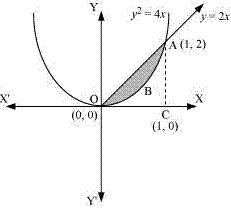The points of intersection of these curves are O(0, 0) and A(1, 2)
We draw AC perpendicular to x - axis such that the coordinates of C are (1, 0).
Area OBAO = Area (ΔOCA) - Area (OCABO)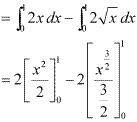= |1 - 4/3|
= |-1/3|
= 1/3 units
Thus, the correct answer is B.